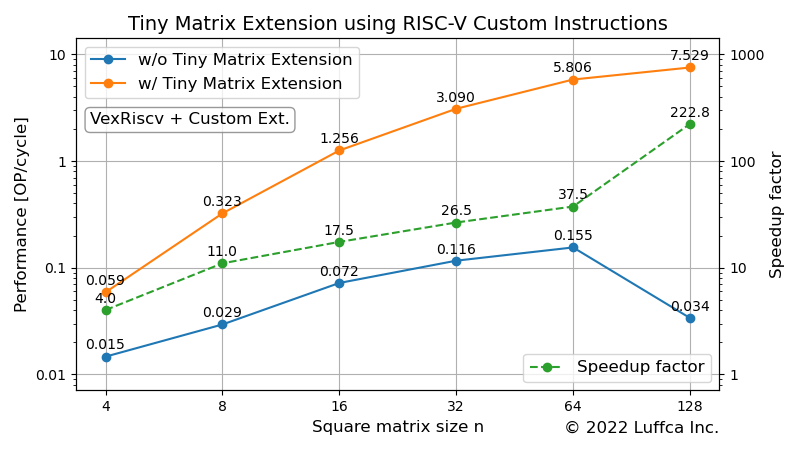# Tiny Matrix Extension using RISC-V Custom InstructionsWe have developed a processor that accelerates matrix multiplication using RISC-V custom instructions, implemented it on an FPGA board, and evaluated its performance.

See related articles here.

## RISC-V Custom Instructions

In RISC-V, custom extensions can be implemented by adding custom instructions, because the instruction code for custom instructions is reserved in advance so that it does not overlap with instruction codes such as MAFDCV, which are extensions specified in the specifications. This also enables the development of DSA (Domain Specific Architecture), a processor designed specifically for a specific domain.

In Gemmini, a DNN accelerator developed at the University of California, Berkeley (UCB), a processor such as Rocket is connected to the accelerator via a RoCC (Rocket Custom Co-processor) interface and controls the accelerator using custom instructions.

In addition, the machine learning (ML) processor introduced in articles such as Building an ML Processor using CFU Playground (Part 1) connects a VexRiscv processor and a circuit for SIMD multiply-accumulate (MAC) instruction through the CFU (Custom Function Unit) interface, and speeds up the inference of ML models using SIMD MAC of custom instruction.

## Tiny Matrix Extension

The tiny matrix extension in this article is not an extension specified in the RISC-V ISA specification like the V (vector) extension, but a custom extension of matrix multiplication using RISC-V custom instructions.

The purpose of the tiny matrix extension is to speed up the matrix multiply code below with lower resource cost.

```// C = AB with A = [n x n], B = [n x n], C = [n x n]
void matmul_int8(int32_t* c, const int8_t* a, const int8_t* b,
const int n) {
for (int i = 0; i < n; ++i) {
for (int j = 0; j < n; ++j) {
int32_t sum = 0;
for (int k = 0; k < n; ++k) {
sum += a[i * n + k] * b[k * n + j];
}
c[i * n + j] = sum;
}
}
}
```

Considering application to ML, the elements of matrix A and B are `int8_t`, and the elements of matrix C are `int32_t`.

In previous article, as part of software development, we created a matrix multiplication kernel based on the RISC-V vector extension (RVV) specification and evaluated its performance using an RTL simulator.

Here, we perform hardware/software co-development, implement it on an FPGA board and evaluate its performance.

## RISC-V Processor with Tiny Matrix Extension

The main specifications of the FPGA board, custom processor, and tiny matrix extension, which is a custom extension, are as follows.

• FPGA board: Digilent Arty A7-35T
• FPGA: XC7A35T
• DRAM: 256 MB
• Custom processor: 32-bit RISC-V
• Base processor: VexRiscv
• ISA: RV32IM + Tiny Matrix Extension
• Operating frequency: 100MHz
• Tiny Matrix Extension
• Hardware interface: CFU
• Software interface: custom instructions
• Square matrix size n: multiple of 4 and less than or equal to 128
• Input data: `int8_t`
• Output data: `int32_t`

The featured image and the table below show the performance of the tiny matrix extension. Performance [OP/cycle] is calculated using the mean of 10 program executions.

Square matrix
size n
Cycles Performance
[OP/cycle]
Speedup
factor
Mean Stdev
16 6522 244 1.256 17.5
32 21211 726 3.090 26.5
64 90297 420 5.806 37.5
128 557096 243 7.529 222.8

Below is an example of the console output when the program is run.

```...

----------------------------------------------------------------
Calculating a (32 x 32) x (32 x 32) matrix multiplication.......
----------------------------------------------------------------

Initializing matrices...
Calculating imatmul with tiny matrix extension...
The execution took 21047 cycles.
The performance is 3.113 OP/cycle.
Calculating imatmul without tiny matrix extension...
The execution took 562829 cycles.
The performance is 0.116 OP/cycle.
Verifying result...
Passed.

----------------------------------------------------------------
Calculating a (64 x 64) x (64 x 64) matrix multiplication.......
----------------------------------------------------------------

Initializing matrices...
Calculating imatmul with tiny matrix extension...
The execution took 90492 cycles.
The performance is 5.793 OP/cycle.
Calculating imatmul without tiny matrix extension...
The execution took 3384030 cycles.
The performance is 0.154 OP/cycle.
Verifying result...
Passed.

...
```

## Summary

We have developed a processor that accelerates matrix multiplication using RISC-V custom instructions, implemented it on an Arty A7-35T from Digilent, and evaluated its performance. For square matrix sizes n of 32 and 64, we achieved speedups of 26x and 37x, respectively, compared to without the tiny matrix extension.

Previous article

Next article